# matching

## Strong matchings and covers ★★★

Author(s): Aharoni

Letbe a hypergraph. A strongly maximal matching is a matching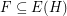so that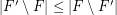for every matching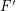. A strongly minimal cover is a (vertex) coverso that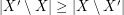for every cover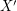.

Conjecture   Ifis a (possibly infinite) hypergraph in which all edges have size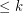for some integer, thenhas a strongly maximal matching and a strongly minimal cover.

Keywords: cover; infinite graph; matching

## Matchings extend to Hamiltonian cycles in hypercubes ★★

Author(s): Ruskey; Savage

Question   Does every matching of hypercube extend to a Hamiltonian cycle?

Keywords: Hamiltonian cycle; hypercube; matching

## Ryser's conjecture ★★★

Author(s): Ryser

Conjecture   Letbe an-uniform-partite hypergraph. Ifis the maximum number of pairwise disjoint edges in, andis the size of the smallest set of vertices which meets every edge, then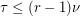.

Keywords: hypergraph; matching; packing